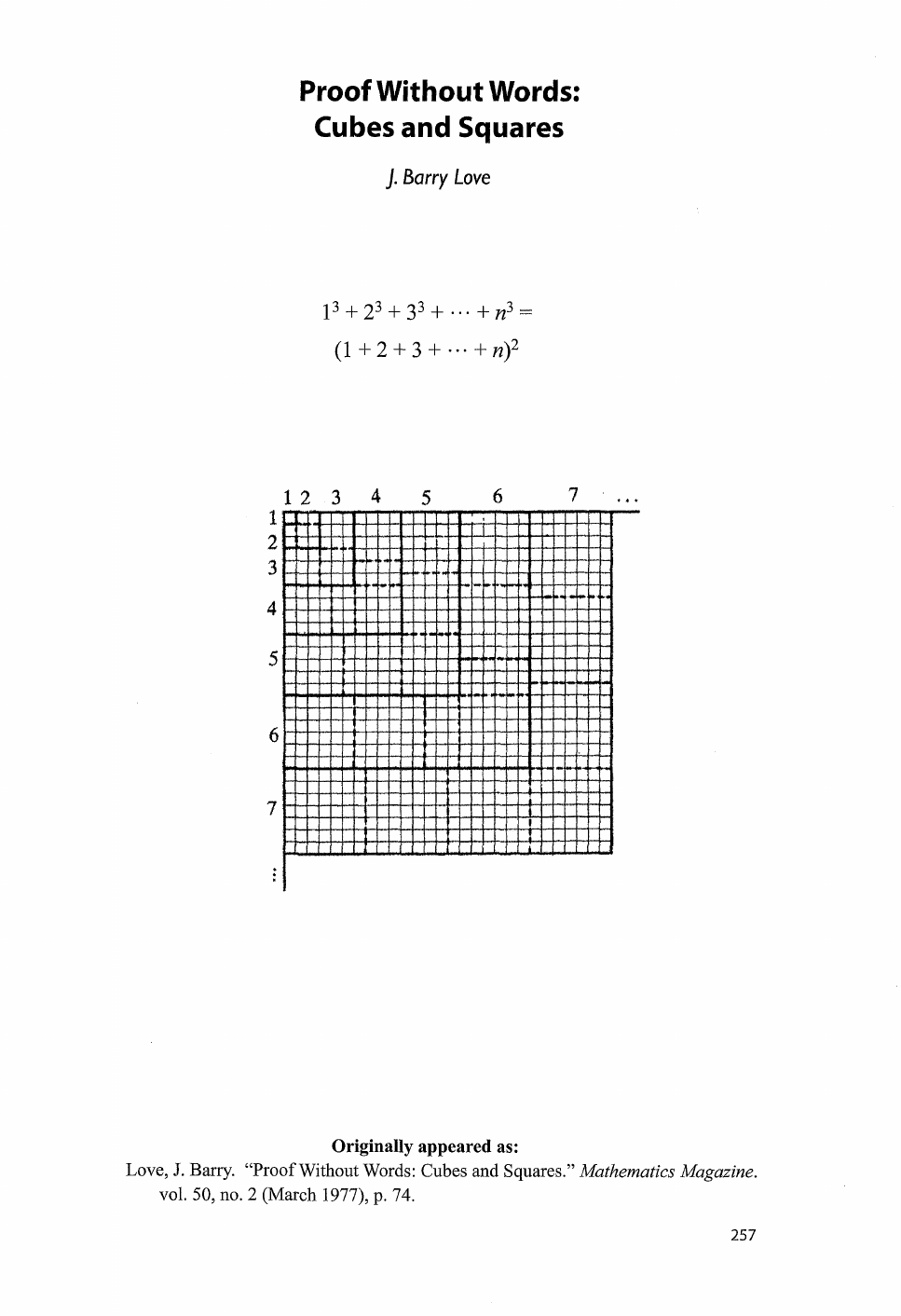First we should try to demonstrate the identity "with words". To do...
The proof "without words" is way simpler than the proof "with words...Proof Without Words:
Cubes
and
Squares
J.
Borry
Love
Originally appeared as:
Love,
J.
Barry. "Proof Without Words: Cubes and Squares."
Mathematics Magazine.
vol.
SO,
no.
2
(March 1977),
p.
74.
The proof "without words" is way simpler than the proof "with words". First we should note that $1^3+2^3+3^3 + ... + n^3$ can be rewritten as $1\cdot 1^2+2 \cdot 2^2+3 \cdot 3^2 + ... + n \cdot n^2$, which geometrically means that we are summing the areas of 1 1x1 square, 2 2x2 squares, 3 3x3 squares ... n nxn squares. The squares can be organized in the following way ![](http://i.imgur.com/KzOnp0j.png) Now we see that if we get all these substructures together we get a square of size $1+2+3+...+n$ ![](http://i.imgur.com/9rQSDvC.png) Thus the area of the square of size $1+2+3+...+n$ is equal to the sum of all the areas of the substructures $$1^3+2^3+3^3+...+n^3= (1+2+3+...+n)^2$$ First we should try to demonstrate the identity "with words". To do so we will need a result that was discovered by Gauss when we was still in primary school: $$1+2+3+...+ n = \frac{n(n+1)}{2}$$ When Gauss was in primary school, he was punished by his teacher due to misbehavior. He was told to add the numbers from 1 to 100. He was able to compute its sum, which is 5050, in a matter of seconds. Gauss noticed that in this kind of sum if we add the first term and the second term, we have $(1 + n)$, $(2 + (n-1))$, $(3 + (n-2))$, and so on. ![](http://i.imgur.com/gyvECva.png) Notice that each pair has a sum of $n + 1$, and we have $\frac{n}{2}$ pairs in total. Therefore, the sum of all the integers from 1 through n is $\frac{(n+1)n}{2}$. With the result above we can rewrite the identity as follows: $$1^3+2^3+3^3+...+n^3 = \Big( \frac{n(n+1)}{2} \Big)^2$$ We are now going to prove the identity above by induction. For $n=1$: $$1^3 = (1(1+1)/2)^2 \Leftrightarrow 1 = 1$$ Now we just need to show that if the result is true for $n$ it will also be true for $n+1$ For $n+1$: $$1^3 + 2^3 + 3^3 +...+ n^3 + (n+1)^3 = \frac{n^2(n+1)^2}{4} + (n+1)^3 \\ = \frac{n^2(n+1)^2+ 4(n+1)^3}{4} = \frac{(n+1)^2(n^2+ 4n+4))}{4}\\ = \frac{(n+1)^2(n+2)^2}{4}$$